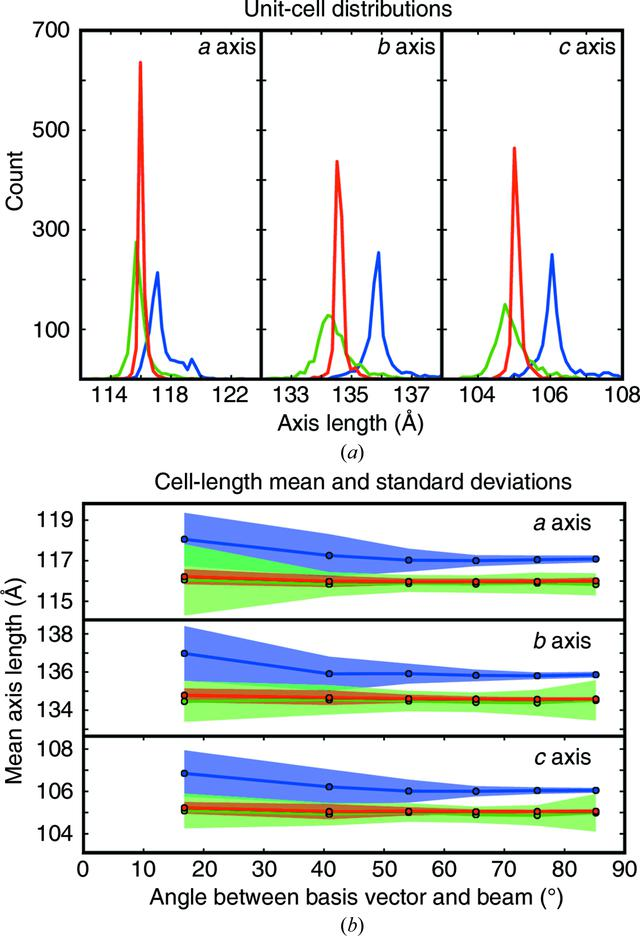disable zoom     view article Figure 6 (a) Unit-cell histograms of 967 Cry3a lattices. The distribution of a, b and c axis lengths is shown. Blue: lattices were indexed and refined separately with no refinement of a detector model. Green: the same crystals after the refinement of 967 crystal models and 967 separate detector models. Red: the same except that the 967 crystal models were refined against a single detector model. (b) The cell-axis lengths from (a) were binned according to the angle of the axis with the beam vector. Bin widths are chosen such that the solid angle subtended within each bin is the same (a basis vector making an angle ρ with the beam will be in bin i satisfying the condition cos−1[1 − (i/6)] ≤ ρ < cos−1{1 − [(i + 1)/6]}, 0 ≤ i ≤ 5). The mean basis vector length for the a, b and c axes is plotted as a line, with one point for each bin at the bin center. The standard deviation among the values is shaded above and below the line. Colors are as in (a). The crystals rotated on average 0.25 and 0.23° during refinement using multiple or single detector models, respectively. The difference between the final models for multiple and single detector models was 0.04°.STRUCTURALBIOLOGY
ISSN: 2059-7983
Volume 74| Part 9| September 2018| Pages 877-894